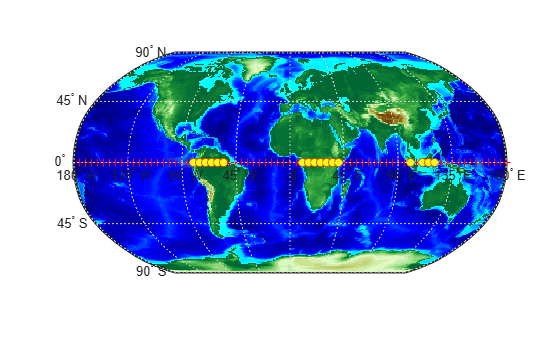# filterm

Filter latitudes and longitudes based on underlying data grid

## Syntax

``````[latout,lonout] = filterm(lat,lon,Z,R,allowed)``````
``````[latout,lonout,indx] = filterm(___)``````

## Description

example

``````[latout,lonout] = filterm(lat,lon,Z,R,allowed)``` filters a set of latitudes and longitudes to include only those data points which have a corresponding value in `Z` equal to `allowed`. `R` can be a geographic raster reference object, a referencing vector, or a referencing matrix.```
``````[latout,lonout,indx] = filterm(___)``` filters a set of latitudes and longitudes, returning indices of the included points in `indx`.```

## Examples

collapse all

Display points along the equator that are above sea level.

First, load elevation raster data and a geographic cells reference object. The raster contains terrain heights relative to mean sea level. Then, specify the coordinates of evenly spaced points along the equator.

```load topo60c lon = (0:5:360)'; lat = zeros(size(lon));```

Create a logical array representing the terrain above sea level. Then, filter the points along the equator to include only the elements that contain `true`.

```topoASL = topo60c > 0; [newlat,newlon] = filterm(lat,lon,topoASL,topo60cR,1);```

Create a map axes object for the world and display the elevation data. Display the all of the points along the equator using red markers. Then, display the points that are above sea level using yellow circles.

```worldmap world geoshow(topo60c,topo60cR,'DisplayType','texturemap') demcmap(topo60c) geoshow(lat,lon,'DisplayType','point','MarkerEdgeColor','r') geoshow(newlat,newlon,'DisplayType','point','Marker','o',... 'MarkerFaceColor','y')```## Input Arguments

collapse all

Latitude values, specified as a numeric array.

Data Types: `single` | `double`

Longitude values, specified as a numeric array.

Data Types: `single` | `double`

Filter, specified as a numeric array.

Data Types: `single` | `double` | `int8` | `int16` | `int32` | `int64` | `uint8` | `uint16` | `uint32` | `uint64` | `logical`

Spatial referencing information, specified as a geographic raster reference object, a referencing vector, or a referencing matrix.

If `R` is a geographic raster reference object, its `RasterSize` property must be consistent with `size(Z)`.

If `R` is a referencing vector, it must be a 1-by-3 with elements:

`[cells/degree northern_latitude_limit western_longitude_limit]`

If `R` is a referencing matrix, it must be 3-by-2 and transform raster row and column indices to or from geographic coordinates according to:

`[lon lat] = [row col 1] * R`

If `R` is a referencing matrix, it must define a (non-rotational, non-skewed) relationship in which each column of the data grid falls along a meridian and each row falls along a parallel. Nearest-neighbor interpolation is used by default. NaN is returned for points outside the grid limits or for which `lat` or `lon` contain NaN. All angles are in units of degrees.

Data Types: `double`

Allowed values, specified as a numeric array or character vector.

Data Types: `single` | `double` | `int8` | `int16` | `int32` | `int64` | `uint8` | `uint16` | `uint32` | `uint64` | `logical` | `char`

## Output Arguments

collapse all

Latitudes of filtered points, returned as a numeric array.

Longitudes of filtered points, returned as a numeric array.

Indices of filtered points, returned as a numeric array.